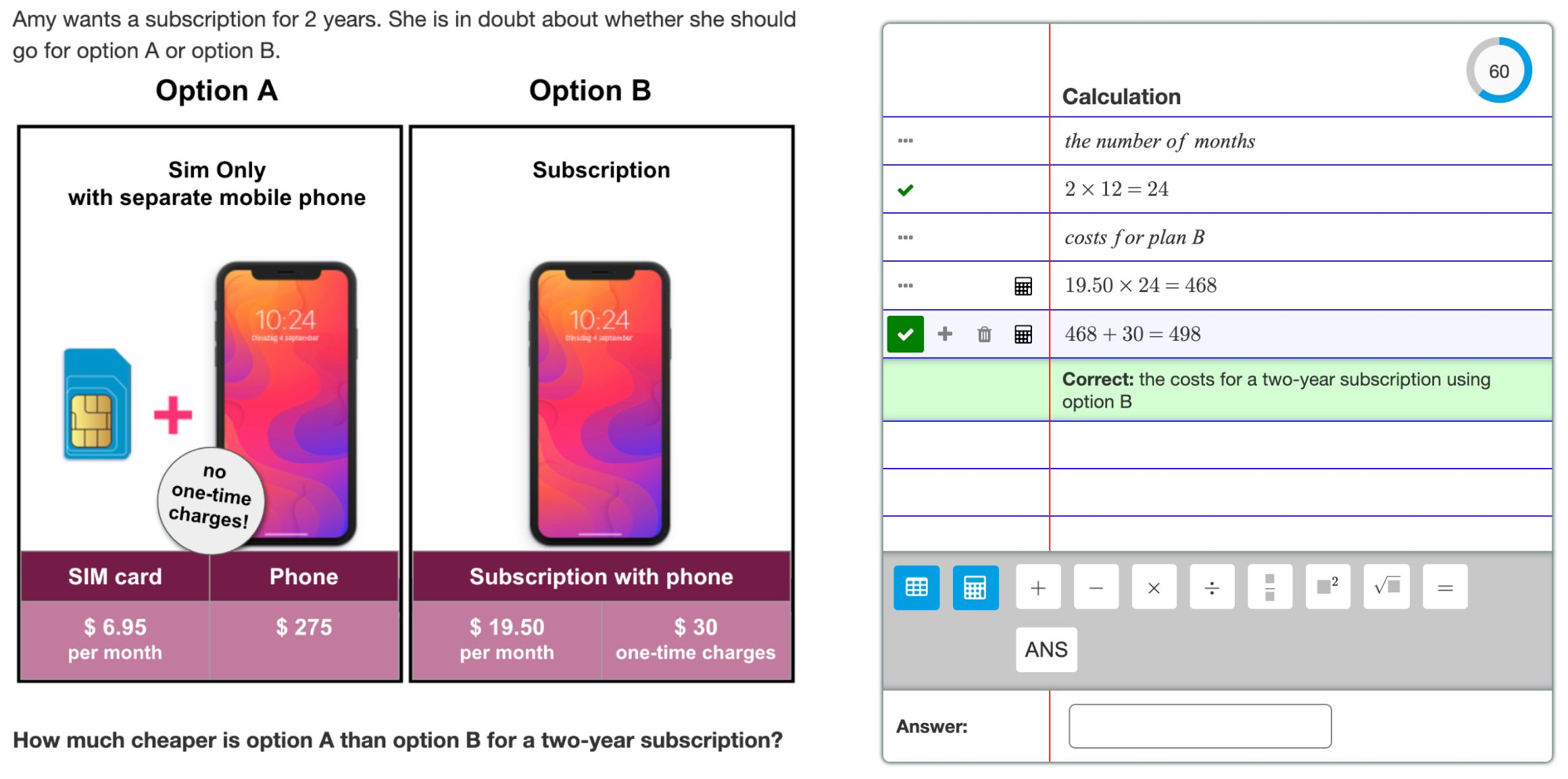# Arithmetic Notebook

## A question type for arithmetic story problems

Story problems (or word problems) are an important part of math education, where students learn to apply mathematics to solve real-world problems. The solution to such problems requires students to go through the following steps:

1. Decompose the problem into partial problems
2. Write a mathematical formulation of each partial problem
3. Use math skills to solve the mathematical problems
4. Interpret the math results to formulate the answer to the real-world problem.

Compared to procedural math problems, such as solving equations or simplifying expressions, story problems require higher-order thinking skills.

Multistep and Arithmetic Notebook question types have built-in support for story problems. Arithmetic Notebook is designed explicitly for arithmetic story problems that do not require variables, formulas, or relations.Figure: An arithmetic story problem with Arithmetic Notebook.

## Features

### Notebook

Students can build their solutions line by line, using either calculations or natural language. Algebrakit evaluates the calculations and neglects the language.

### Built-in calculator

With the built-in calculator, students do not have to retype the calculations in their calculator. This prevents students from being distracted by procedural tasks and keeps the focus on higher thinking skills.

### Positive feedback

Algebrakit adds a checkmark to each correct line. There is no negative feedback if Algebrakit cannot identify a line as an intermediate step. This stimulates students to use the input field as a notebook, where they are free to express their thoughts.

The progress indicator is an additional feature to assure students they are progressing.

### Conceptual feedback

Students receive conceptual feedback, such as descriptions of what the calculations represent or hints about what to calculate (not how).

### General Features

Like the other question types, Arithmetic Notebook can run in assessment mode to prevent students from seeing evaluation results, hints, or error feedback. This information is available during the review after the student submits the questions or tests.

Arithmetic Notebook also supports random questions.